Contents

Spreadsheets are used to store and manipulate data. Spreadsheet data will usually be organized as a table, given that spreadsheet itself is one giant array of empty table cells. Table cells can contain a value (text or number) or a formula. Formulas calculate new values, usually by referencing existing values, and parameters of those calculations can be easily changed by user.

These simple concepts can then be used to perform either basic tasks, i.e. you can use your spreadsheet as a tape calculator.

On the other hand, strength and scalability of a spreadsheet concept enables ordinary users without programming knowledge to, basically, create and manage rational databases and write programs.

This is what an empty spreadsheet looks like in Microsoft Excel: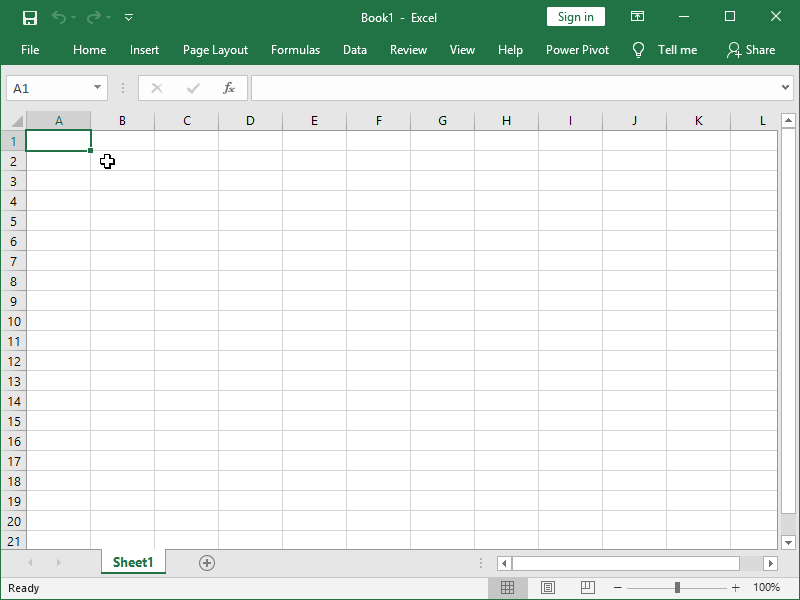You are now on cell A1, as indicated by highlighted border around cell A1.

A1 is than the address of your cell – i.e. your cell is located in column A of XFD columns and row 1 of 1048476 rows.

Think of a cell as of container for some of your data. If you want to add something to cell A1, or change existing data, you can enter into the cell by double-clicking it.

You can type some text in that cell. If you do, it will be aligned to the left.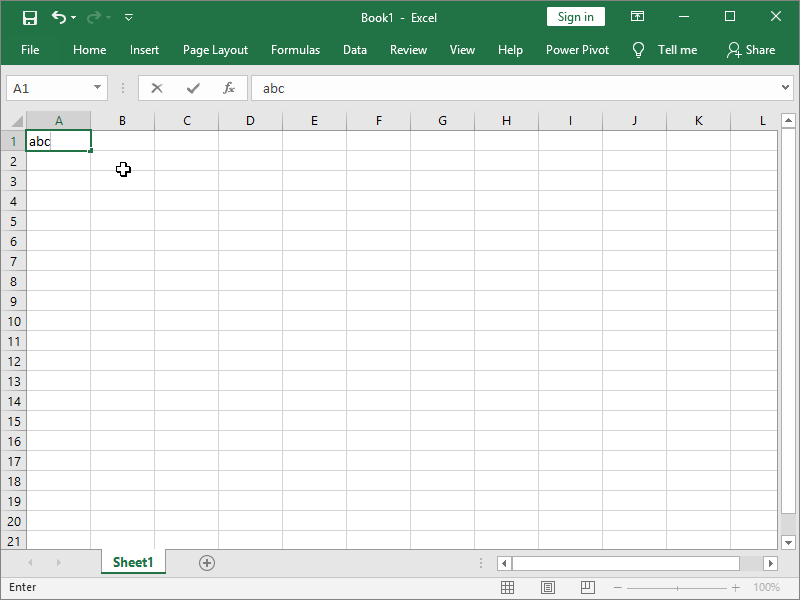You can type a number in that cell. If you do, it will be aligned to the right.

You can also do this in formula bar – you can change address of the cell you are editing on the left of the formula bar if you want, and contents on the right: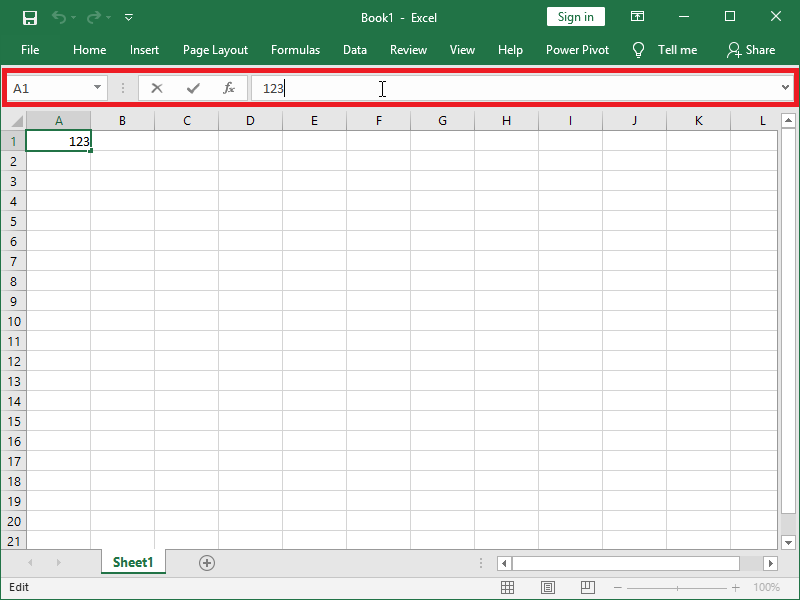You can select multiple cells at once. If you right-click click on your selection you will get a pop-up menu with options to manipulate all those cells at the same time. For example, you can delete contents of all selected cells.

You can select one whole column in you left-click column name. If you click on column name A, you will select column A. If you click on column name A and drag right, you will be able to select multiple columns at once:

It works the same with rows.

Width of your columns and height of your rows is irrelevant for your data, it’s purely visual. If you want to change the width of column A, position your mouse between the names of columns A and B, click and drag. You can change row height the same way.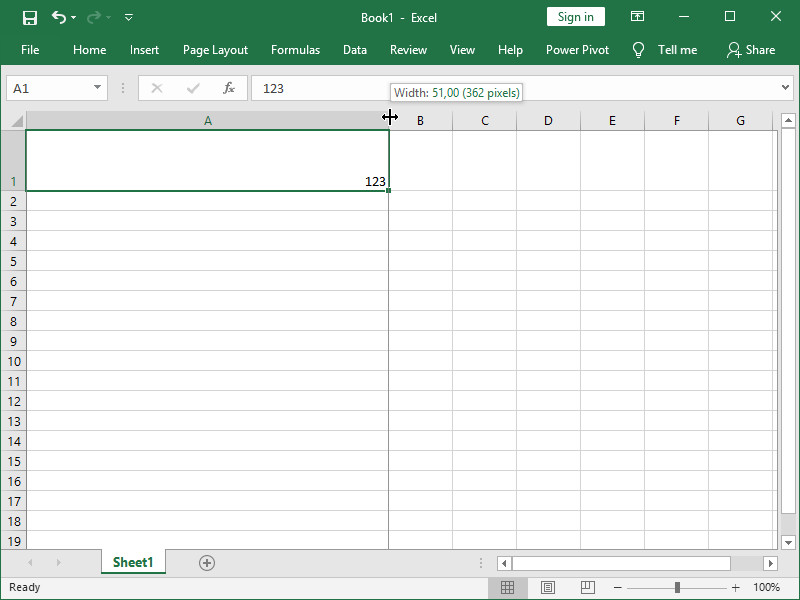This array of cells organized in rows 1 of 1048476 and columns A to XFD is called a spreadsheet, worksheet or just sheet. Here, it’s called “Sheet1”, but we can also rename it after we double-click it: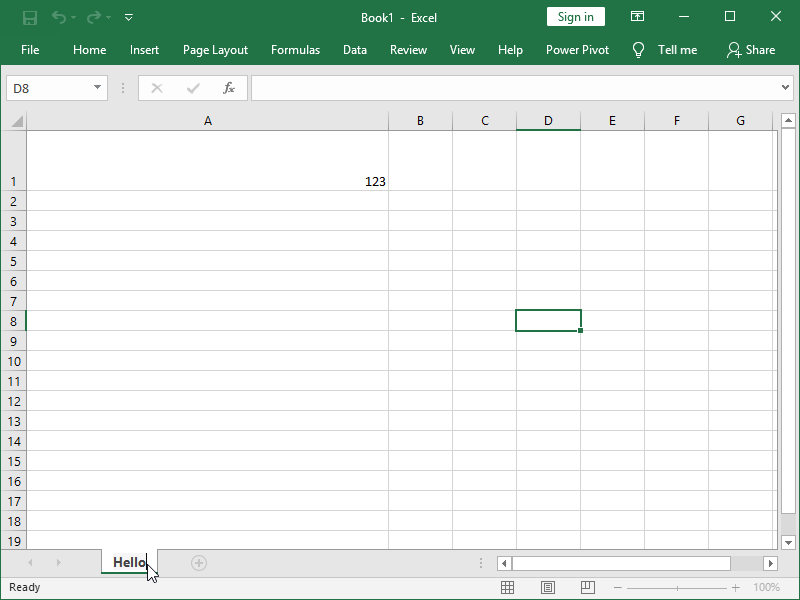By clicking “New sheet” button, you can also add new sheets: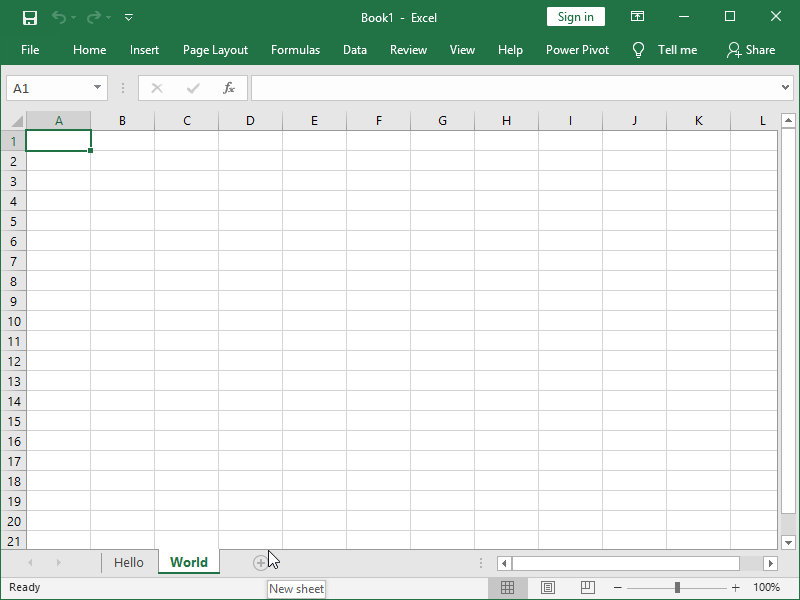If we wanted to do so, we could also duplicate any existing sheet by right-clicking its name and selecting “Move or Copy…”: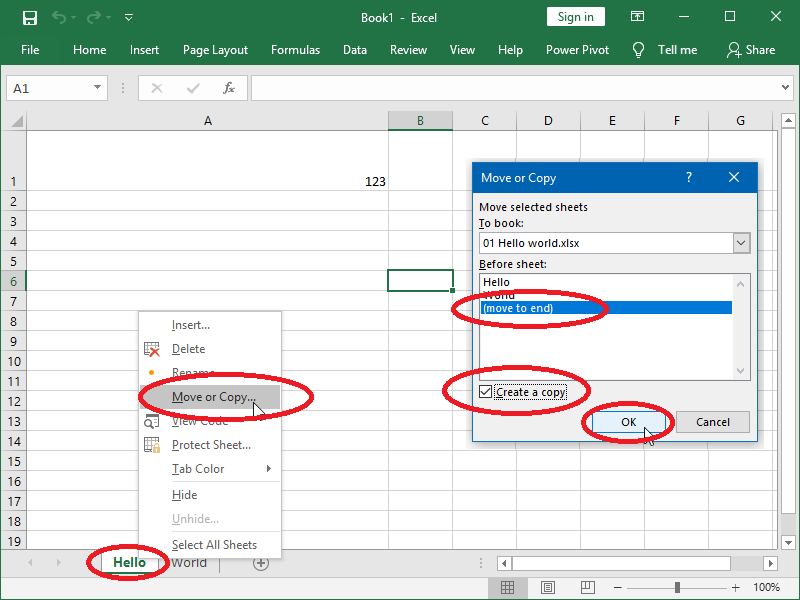One or more of these sheets combined are called workbook, or just book.

You can already see at top center of the window that your workbook was automatically named “Book1”. You can rename your workbook by saving it with save button located top left of the window: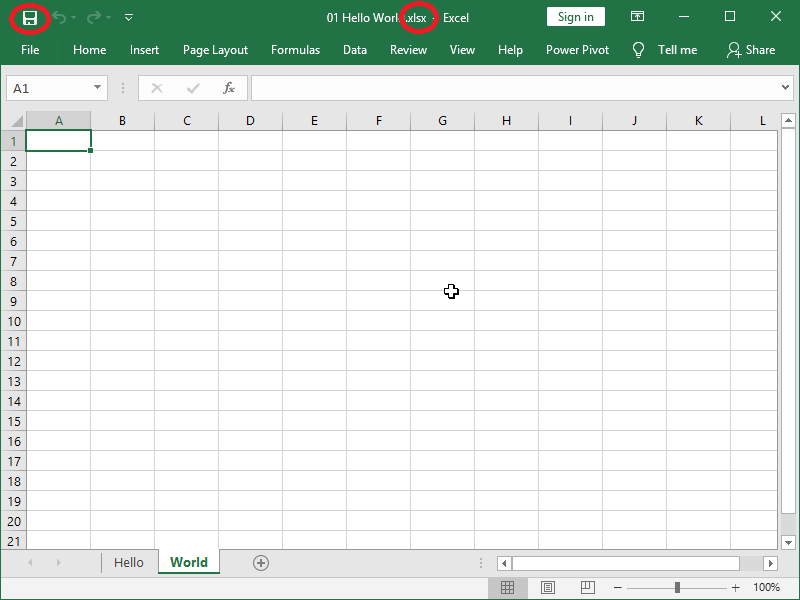We have now named our workbook “01 Hello world”. Excel workbooks should be saved with file extension “.xlsx”.

Note that Excel can open and read and save files with many different types of extension, such as legacy spreadsheets with extension “.xls”, spreadsheets of other programs such as LibreOffice with extension “.ods”, XML encoded files, web pages and text files. Nevertheless, in order to avoid issues it’s recommended that you save any file you crate of modify with Microsoft Excel as “.xlsx”.

### How to use formulas in Excel?

A table cell can contain a value (text or number) or a formula.

If a cell contains a formula, it actually contains both that formula and a value calculated by that formula. While we are on a cell, we will see that calculated value. In the formula bar, we will see the formula in that cell. Here is how that looks: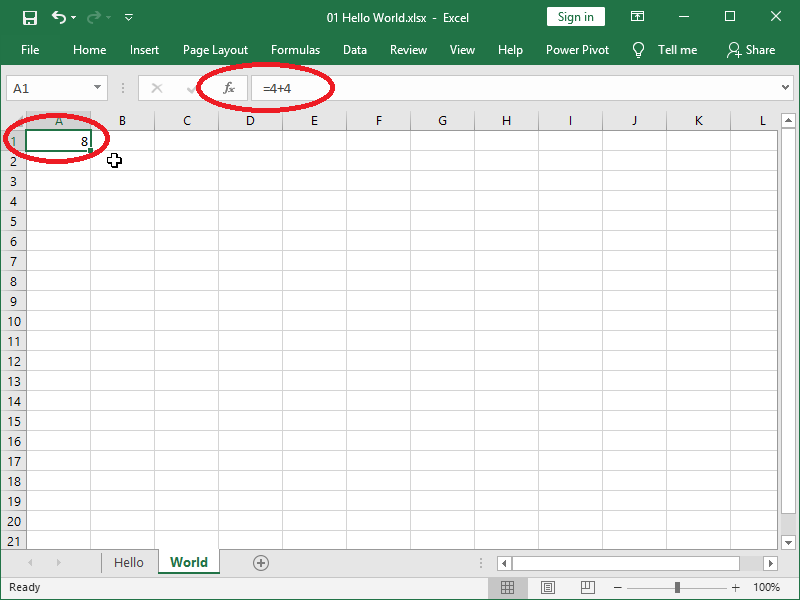But, if we double click on the cell and enter it, we will also be able to see and change the formula. Here is how that looks: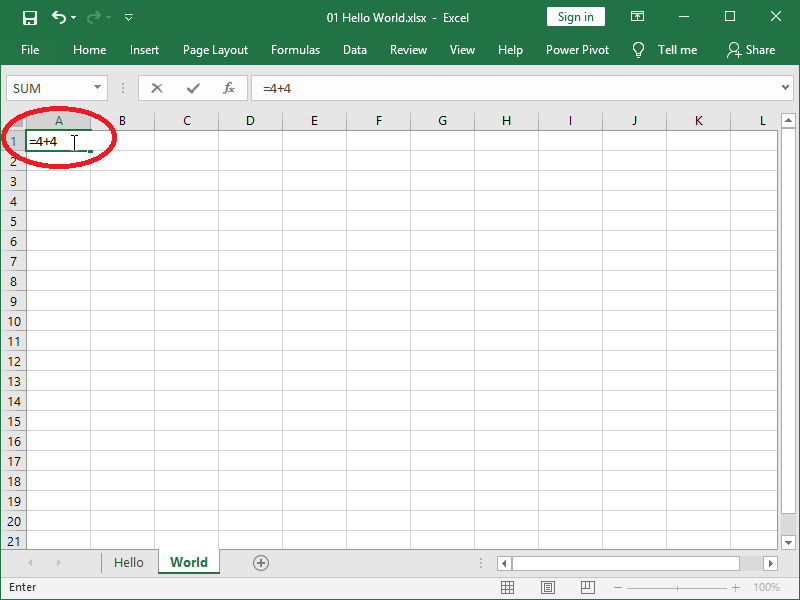We can also refer to another cell with formula, including empty cells, and those will be interpreted as zero.

Referred cell doesn’t have to be in the same sheet. For example, in order to refer to cell A1 in sheet “Hello” we have to fist invoke the name of that sheet followed with exclamation mark [!] and then cell address: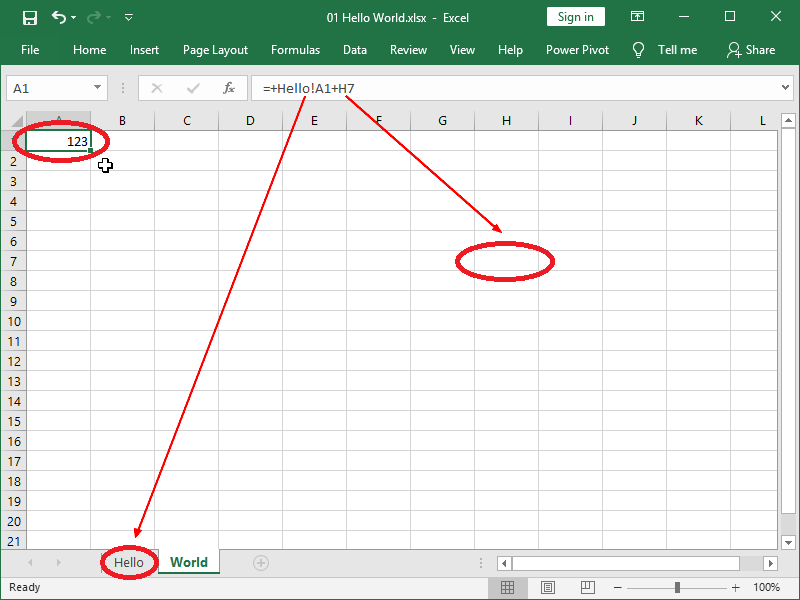We can start writing any formula by writing equals sign [=], or even writing plus sign [+] with Excel automatically adding equals sign in that case.

Formula can contain:

• a value,
• the address of a cell (meaning: a value stored in the cell we are calling, whether it is just a value or value calculated using some other formula),
• a function,
• any combination of the above.

Basic mathematical operations we can perform with values are addition [+] subtraction [-], multiplication [*], division [/] and exponentiation [^].

Following example shows us performing basic mathematical operations with formulas that are referencing neighboring cells, and are also containing values.

When editing formula directly in the cell, Excel automatically highlights referenced cells visible on screen: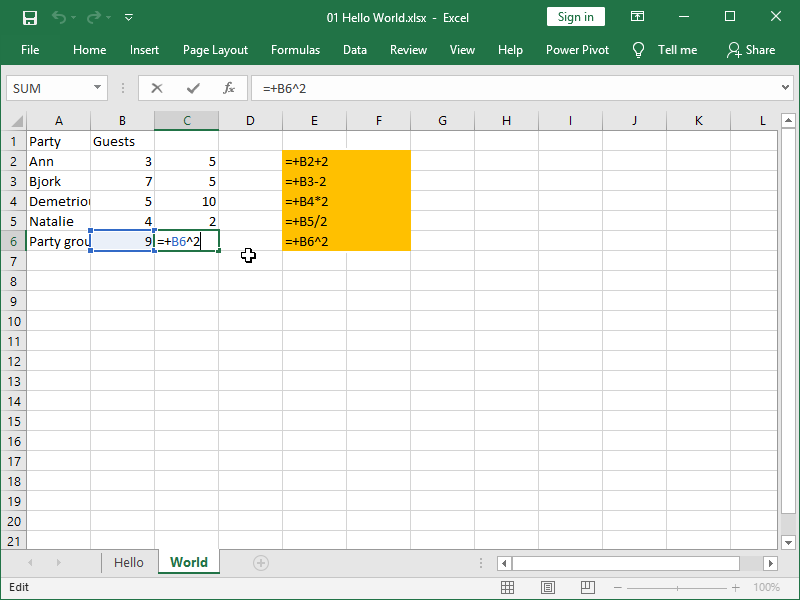Here, we were referring to the values in cells in column B and performing different mathematical operations on them with normal or relative references.

If we copy or drag the formulas containing relative references, for example, if we copy formulas from column C to column D, cells referred will also move.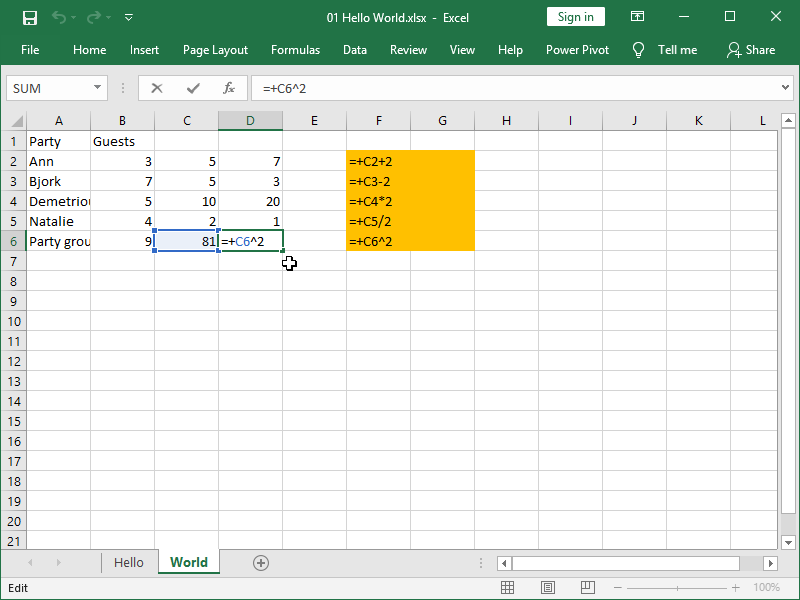If we want to avoid that, we can use locked or absolute references. That type of reference, in addition to column and row address, aslo contains dollar [\$] signs.

This is how we write the formula with absolute reference: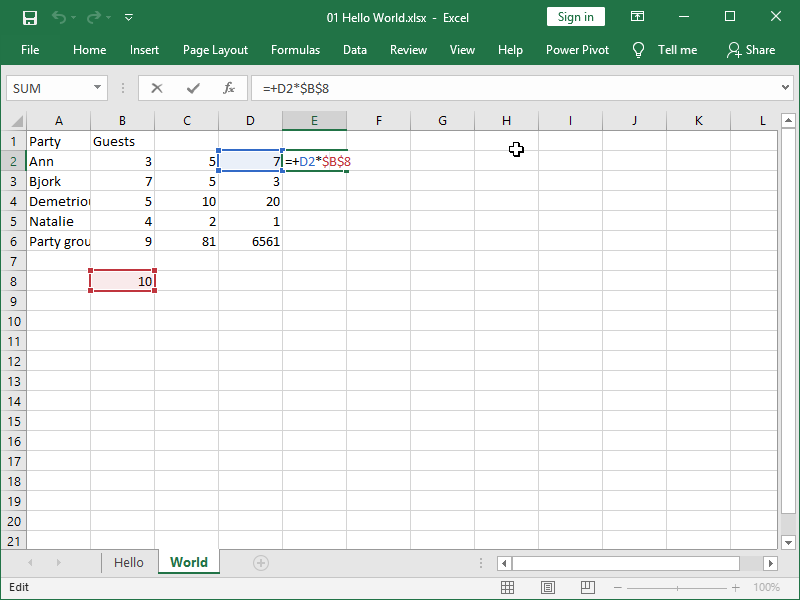Even if we now drag that formula down, we will be still referring to cell B8 in all of the new formulas: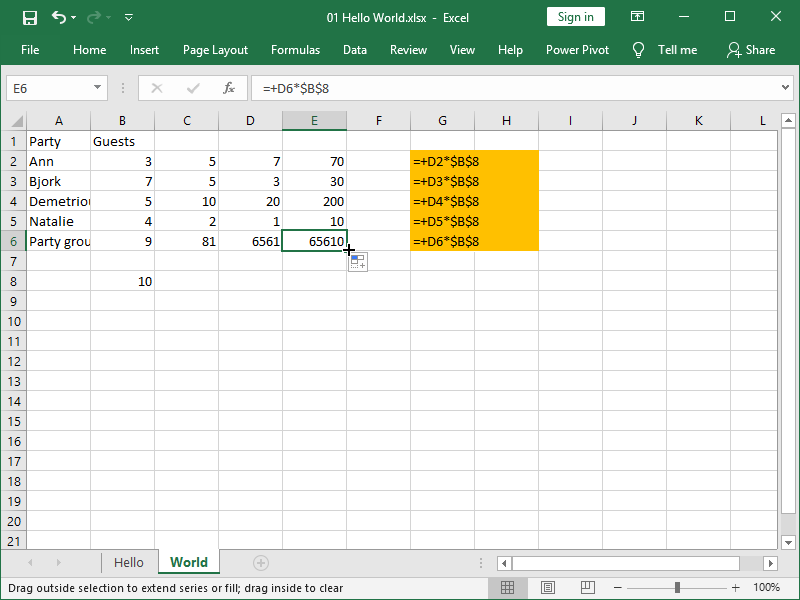We are also able to lock only the column we are referring to by placing dollar sign in from of a column reference, but not row reference, i.e. if we write \$B8. This works the other way too, i.e. we can write B\$8.

Dollar sign has no effect on calculations themselves, and absolute references are in principle only useful if we are copying or dragging formulas and want to ensure that the same cell/column/row is always referenced.

### How to use functions in Excel?

In addition to the basic mathematical operations, we can also use huge number of functions available in Excel in our formulas. You can see complete list of those functions under Formulas > Insert Function, together with the short description of each function: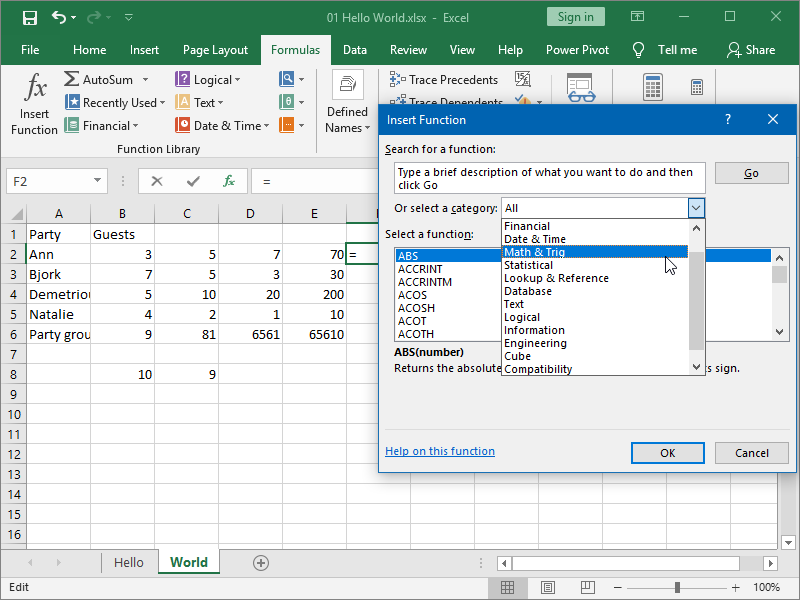Alternatively, if you insert the function by typing its name in the cell directly, you also get some help from Excel: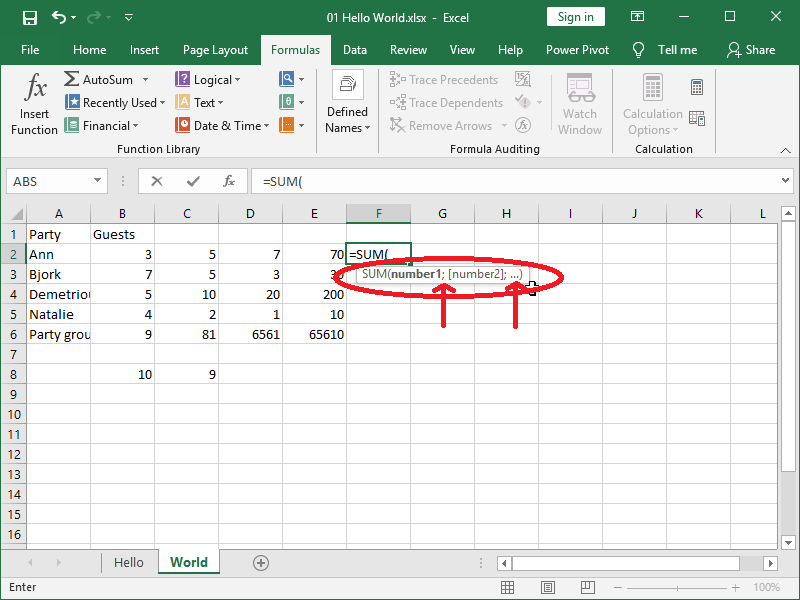Here you can see that, in this example of the SUM function:

• the function should be called by name with arguments between parenthesis, i.e. SUM ()
• you can aggregate however many numbers you desire, as indicated by three dots […]
• different numbers you are aggregating should be separated by semicolon [;].

In functions, you can also:

• reference cells
• reference ranges of cells
• call other functions.

As mentioned before, you can also combine any number of functions, cell references and numbers in formulas.

Here is one example of all of those principles: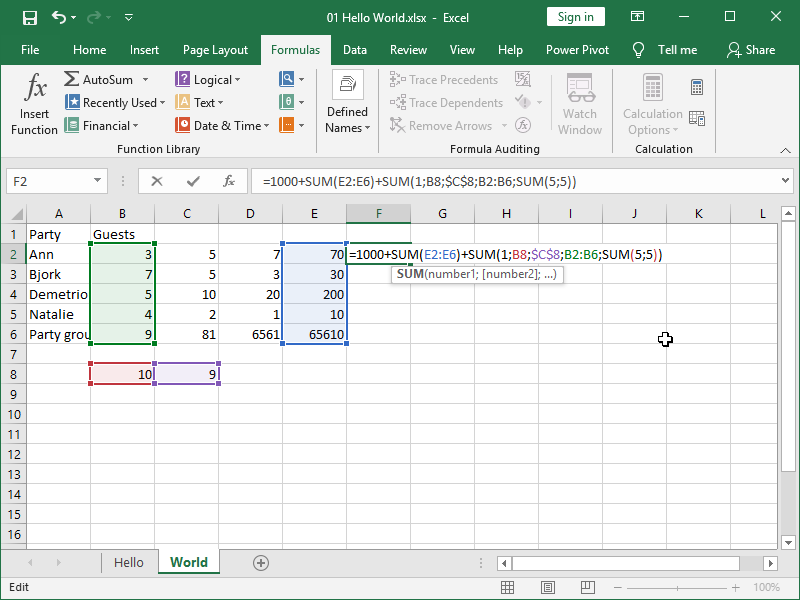As you can see, Excel also helpfully highlights cell ranges the same way it does for cells you are referring to.

Purpose of a function is to make your life easier, i.e. it is easier and less prone to errors to write formula such as =SUM(E2:E6) than formula such as =E2+E3+E4+E5+E6.